# Comparing and ordering large multi-digit integers

### Comparing and ordering large multi-digit integers

In this lesson, we will learn:

• To compare integers—positive and negative whole numbers—up to millions place values
• To order a list of integers—positive and negative whole numbers—up to millions place values

Notes:

• When comparing numbers, we use the symbols: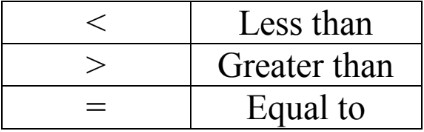• For integers, use a number line to understand which numbers are bigger or smaller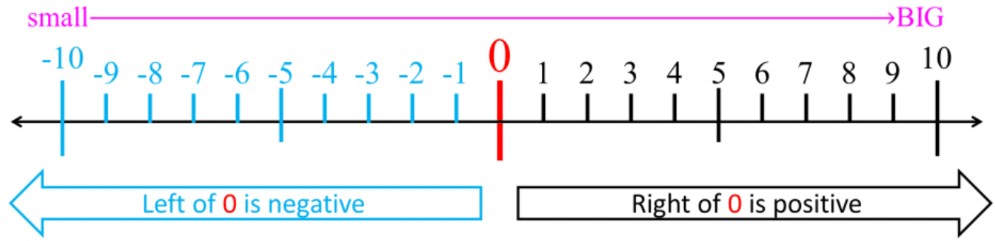• Smaller numbers are to the left; bigger numbers are to the right
• Ex. Look at the pairs of opposite numbers: -5 < -2 vs. 5 > 2

• For negative numbers: the bigger the negative, the smaller the value (i.e. more negative means less value)• The same rules apply for comparing multi-digit integers:
• Ex. Compare the number -5000 with: 6000, 5000, 0, and -6000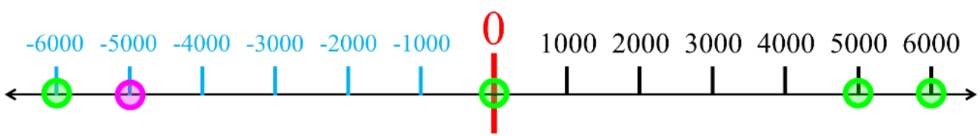• -5000 < 6000
• -5000 < 5000
• -5000 < 0
• -5000 < 6000

• There are two ways to order a list of numbers, including multi-digit integers:
• From least to greatest (smallest to biggest)
• most negative to least negative
• From greatest to least (biggest to smallest)
• least negative to most negative
• Ex. -6000 < -5000 < 0 < 5000 < 6000

#### Lessons

• Introduction
Introduction to Comparing and Ordering Large Multi-Digit Integers:
a)
How to compare two large multi-digit integers (positive and negative)

b)
How to order two large multi-digit integers (positives and negatives)

• 1.
Comparing integers on a number line
Draw dots on the number line for each integer. Circle the number that is bigger.
a)
-4 and 2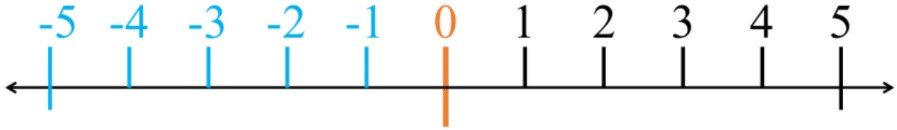b)
-153 and -163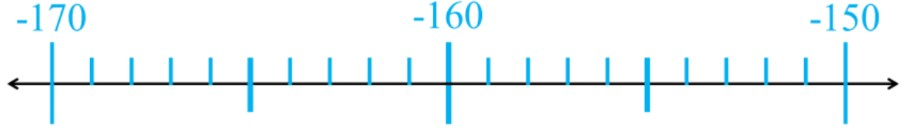c)
-72 000 and -78 000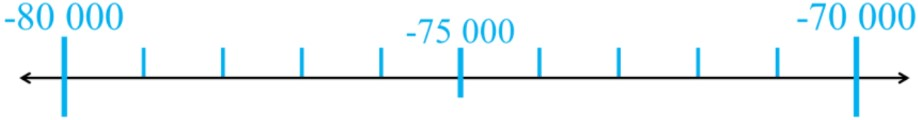• 2.
More or less negative integers
Circle the integer that is more negative. Write "less" or "greater" under each number.
a)
-12 vs. -13

b)
-2867 vs. -2495

c)
-3,829,001 vs. -3,840,756

• 3.
Ordering multi-digit integers: least to greatest
Order the integers from least to greatest. Use the less than symbol (<).
a)
-14, 0, -25

b)
-956, -487, -1023

c)
-51 247, -41 247, -61 247

d)
-418,754,629 , -147,856,023 , -482,739,165

• 4.
Ordering multi-digit integers: greatest to least
Order the integers from greatest to least. Use the greater than symbol (>).
a)
-5, 1, -3

b)
-1747, -7417, -4177

c)
-985,176 , -593,229 , -697,047

d)
-34,867,921 , -43,532,107 , -33,124,689

• 5.
Writing a multi-digit integer within a range
Write an integer between each pair of integers in the following scenario:
a)
One year, the weather averages in the month of October had the highest temperature of 6°C and the lowest temperature of -8°C.

b)
During a deep-sea exploring expedition, a diver was at 157 feet below sea level (-157 ft). A few minutes later, the diver was pulled up and is now at 132 feet below sea level (-132 ft).

c)
There are no popular movies in theatres this week. On Monday, the theatre company reported a loss of -$127,613. By Wednesday, there was a total loss of -$540,859 for the week already.